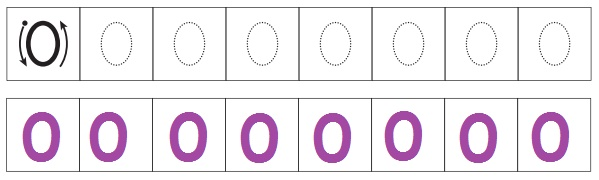Home | | Maths 1st Std | Zero

# Zero

Teacher's note: Teacher can use the spindle board of SLM kit for enhancing the concept of Zero. 48

Zero

Travel through

I am the Hero My name is Zero

Three bits of paper lying on the floor,

pick one up, put it in the bin;

Look at the floor now, two are left.

Two bits of paper lying on the floor,

pick one up, put it in the bin;

Look at the floor now, one is left.

One bit of paper lying on the floor,

pick it up, put it in the bin;

Look at the floor now, there is no paper left.

LearnO is also a number.

Teacher's note: Teacher can use the spindle board of SLM kit for enhancing the concept of Zero. 48

PracticePleasure time

1. Count and write the number of butterflies.2. Count and write the number of pencils.3. Count and write the number of tomatoes.Tags : Numbers | Term 1 Chapter 2 | 1st Maths , 1st Maths : Term 1 Unit 2 : Numbers
Study Material, Lecturing Notes, Assignment, Reference, Wiki description explanation, brief detail
1st Maths : Term 1 Unit 2 : Numbers : Zero | Numbers | Term 1 Chapter 2 | 1st Maths